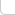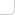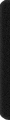# 某岛

… : "…アッカリ～ン . .. . " .. .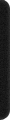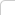July 4, 2012

# SRM 484

### Brief description:

Problem 250. RabbitNumber (兔子数 …

( .. . 1 <= l, r <= 10^9 .. ) Problem 550. PuyoPuyo 一个堆栈, 可以往里面放 4 种颜色的球，当栈顶连续 L 个球颜色相同时，消去这 L 个球。 问往里面放 n 个球的话有多少种放法使得栈空。 ( . ..n <= 1000, L <=10 .. .) Problem 950. NumberMagic (猜数游戏。。 Taro 在心中想了一个 1～n 之间的数字 x，Hanako 每次可以询问一个 m 大小的集合。 Taro 返回 x 在 / 不在 其中。。。问至少多少次询问一定可以猜出这个数。

### Analysis:

Problem 250. RabbitNumber
。貌似暴力 DFS() 可过。。
( 可以推出兔子数就是满足 x^2 不产生进位的数。。(。。反证立得。。一旦产生进位则必然有所损耗。。。
( 则每一位只能是 [0, 3] 。。并且前缀一定也是兔子数。。。

Problem 550. PuyoPuyo

Problem 950. NumberMagic (猜数游戏。。

。。也就是对任意的 i, j，都有 {Si} 不同于 {Sj} 。。。（ {Si} 表示包含 i 的询问集合。。

… 然后这个题很容易让人往不对的地方想 。。。

f(n, m) = f(n, n – m)。（因而该函数类似组合数。是对称的、单峰的、不过是凹的。。
f(2^k, 2^(k-1)) = k 。

```int f(LL x){
int s = 0;
while (x) s += x%10, x/=10;
return s;
}

class RabbitNumber{
public:
int L, R, res;
void dfs(LL x, LL s){
if (s > 14 || x > 1e9) return;
if (L<=x && x<=R)
if (s*s==f(x*x)) res++;
for (int i=0;i<4;i++)
dfs(x*10+i, s+i);
}

int theCount(int l, int r){
L = l, R = r, res = 0; if (R==1e9) res++, R--;
for (int i=1;i<4;i++) dfs(i, i);
return res;
}
};
```
```const int N = 1009, M = 10009;

int dp[N][N];

class PuyoPuyo {
public:
int theCount(int l, int n) {

RST(dp), dp = 1; REP(i, n) {
INC(dp[i+1][l-1], pdt(4, dp[i]));
REP_1(j, min(n-i, (l-1) * i)){
INC(dp[i+1][j-1], dp[i][j]);
INC(dp[i+1][j+(l-1)], pdt(3, dp[i][j]));
}
}

return dp[n];
}
};
```
```.. .
int n, m;

LL C(int n, int m){
LL res = 1; REP_1(i, m) res *= (n - i + 1), res /= i;
return res;
}

bool check(int k){
LL r = (LL) m * k; int n = ::n, t;
for (int i=0;i<=k&&n&&r>=0;++i){
t = min(C(k, i), (LL) n);
n -= t, r -= (LL) i * t;
}
return r >= 0 && n == 0;
}

class NumberMagic {
public:
int theMin(int n, int m) {
m = min(m, n - m), ::n = n, ::m = m;
int l = 0, r = n - 1;
while (l < r){
int k = (l + r) >> 1;
if (check(k)) r = k;
else l = k + 1;
}
return l;
}
};
```

If it is possible to place a total of M*K numbers on K cards in a way that satisfies the original condition, it is possible to place exactly M numbers on each of K cards in a way that satisfies the original condition.

。下面给出构造方案。。（ k 太小的话构造不出满足条件的解。。

。。考虑到 “用料最省” 的原则。。（同时又要满足最开始所述的条件。。。
。。0 次 的只能有 1 个数字。。（如果有 2 个则无法区分开。。
。。1 次 的只能有 k 个数字。。（每张牌占一个。。
。。2 次 的只能有 C(k, 2) 个数字。。。。（。。同理。。
。。。。。
。。K 次 的只能有 C(k, k) 个数字。。。

。。K 次 的只能有 1 个数字。。（如果有 2 个则无法区分开。。
。。K-1 次 的只能有 k 个数字。。（每张牌占一个。。
。。K-2 次 的只能有 C(k, 2) 个数字。。。。（。。同理。。
。。。。。
。。0 次 的只能有 C(k, k) 个数字。。。

（。化简后。。逻辑上理解即取反。。。

。这个区间是否可以包含 mk。。。

（。。证明不能。。